Next: 5. Conclusions/References Up: 4. DWE Instrumentation: Ultrastable Previous: 4.3 USO frequency characteristics

## 4.4 USO Test Program

At this writing, only results from the EM test program are available. The DWE USO Electrical Model is shown in Fig. 9, prior to integration with the aluminum housing removed.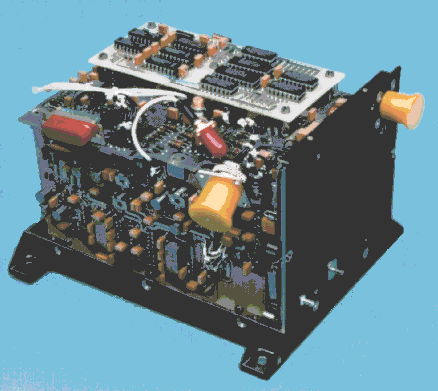Figure 9: The DWE-TUSO engineering model. The input power connector and grounding studs are on the right side. The remaining ports facing the viewer are (from left) test connector (red, elongated), the 10 MHz RF output (red, round), and the telemetry output connector (yellow).

A USO test assembly (block diagram: Fig. 10), developed at the University of Bochum, is used at unit level to determine USO frequency characteristics. Of particular interest is the frequency stability of the USO on all time scales. It is also necessary to characterize the repeatability of the long-term drift of the USO in order to correct for this effect under the conditions of the Huygens descent.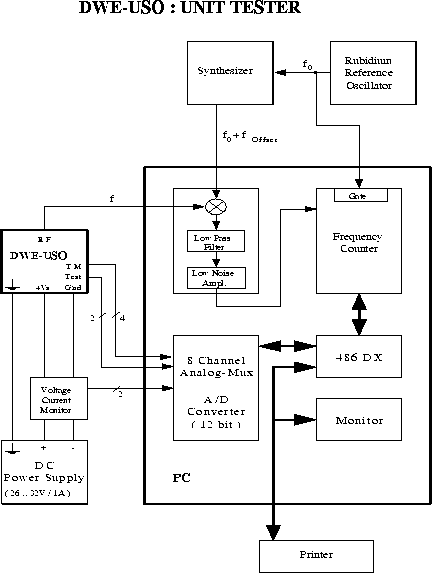Figure 10: Block diagram of the test assembly.

The frequency measurement procedure is based on a comparison of the test frequency f (DWE-USO) with a reference signal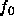(rubidium reference oscillator) whose frequency stability is better than or equal to that of the test signal. Using a synthesizer, the reference frequency is offset from the nominal frequency of the test objectby a known amount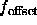. The difference frequency of the two signals is generated with the help of a mixer and then measured by a frequency counter. Following a fully automated procedure, both the long-term and short-term stabilities of the test object are determined by recording the difference frequency for an appropriately long interval (nominal test run: 3 hours). The reference and offset frequencies, as well as the integration and sample times can be adjusted as necessary with respect to their default values for each individual run.

The number of single measurements is defined by the ratio of the adjusted total measurement time to the mean duration of the single measurement cycle. A single measurement cycle consists of the adjusted fixed integration time and the counter register read-out time. The read-out phase requires about 20 ms. The mean duration of one measurement cycle is thus about 1.02 sec for an integration time of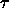= 1s, and 10.02s for= 10s, respectively.

The absolute frequency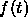and the Allan variance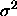are then derived as follows: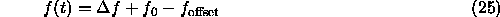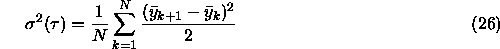where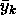=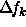/,=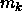/, andis the k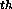cycle count over the integration time.

Examples of short-term stability results from a typical test run during the unit level TUSO-EM program are displayed in Fig. 11. Running measurements of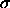, defined by (26) are made for integration times= 1 s and 10 s. Each point in Fig. 11 represents 100 such measurements. The mean values of the Allan deviation in this case were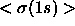= 1.8·10E-11 and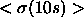= 5.5·10E-12, respectively.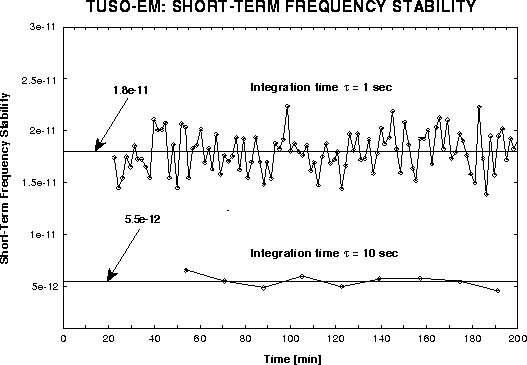Figure 11: Short-term frequency stability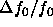of the DWE-TUSO Electrical Model.

The behavior of the USO output frequency as a function of time from switch-on is shown in Fig. 12 for two test models, one with and one without a temperature-compensating magnetic control loop. These tests were performed at an ambient temperature of + 25°C.

After reaching its asymptotic value, the output frequency of both models stays within a band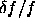<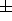2·10E-10. The model without magnetic compensation was found to require too much time to warm up and also exhibited undesireable variations in asymptotic output frequency with temperature. Significant improvement was achieved by implementation of a magnetic control loop, which exploits the sensitivity of the rubidium hyperfine transition to the strength of the magnetic field in the rubidium cell. The strength of the magnetic field applied to compensate the thermal drift is determined via a feedback loop using the thermal sensor in the crystal oscillator. Preliminary results from recent thermal vacuum tests (FM Test Program) over an ambient temperature range from - 30°C to + 60°C indicate that: (a) the USO warm-up time is always less than 30 minutes, and (b) the variation in mean asymptotic frequency is less than 2·10E-10.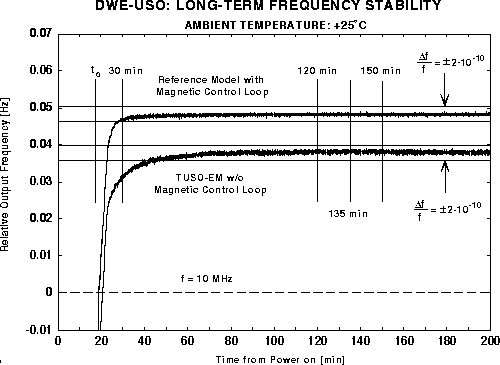Figure 12: USO output frequency as a function of time from switch-on.

DWE Homepage - For further information contact r.dutta-roy@freenet.de